# Understand Adding and Subtracting Fractions with Different Denominators and Mixed Numbers

In this worksheet, students will be asked to add and subtract fractions with different denominators and also add mixed numbers.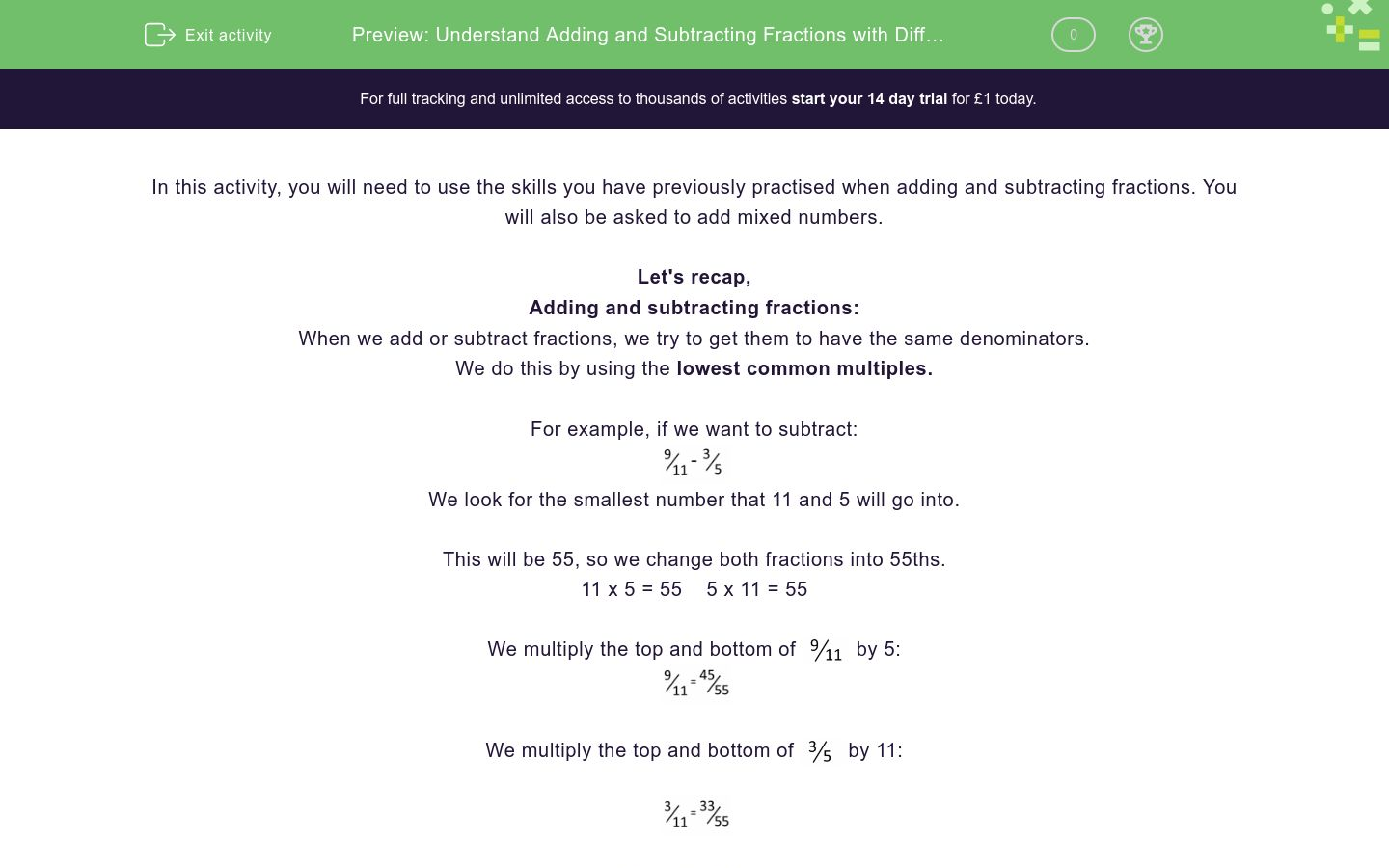Key stage:  KS 2

Curriculum topic:   Number: Fractions, Decimals and Percentages

Difficulty level:### QUESTION 1 of 10

In this activity, you will need to use the skills you have previously practised when adding and subtracting fractions. You will also be asked to add mixed numbers.

Let's recap,

When we add or subtract fractions, we try to get them to have the same denominators.

We do this by using the lowest common multiples.

For example, if we want to subtract:We look for the smallest number that 11 and 5 will go into.

This will be 55, so we change both fractions into 55 ths.

11 x 5 = 55    5 x 11 = 55

We multiply the top and bottom ofby 5:We multiply the top and bottom ofby 11:So now we have:Answer =Adding or subtracting mixed numbers involves 4 steps.

What is?

1. Add or subtract the whole numbers

3 + 7 = 10

2. Add or subtract the fractions.

The lowest common multiple is 45, firstly, convert both fractions to 45ths.3. Can you simplify the answer?

Yes! Both numbers can be divided equally by4. The final piece of the puzzle! We add the whole number from Step 1 and the fraction from Step 3 together.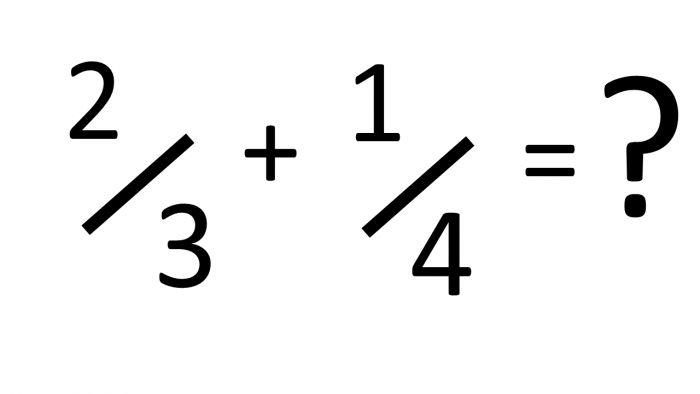Can you choose the correct answer for the following calculation?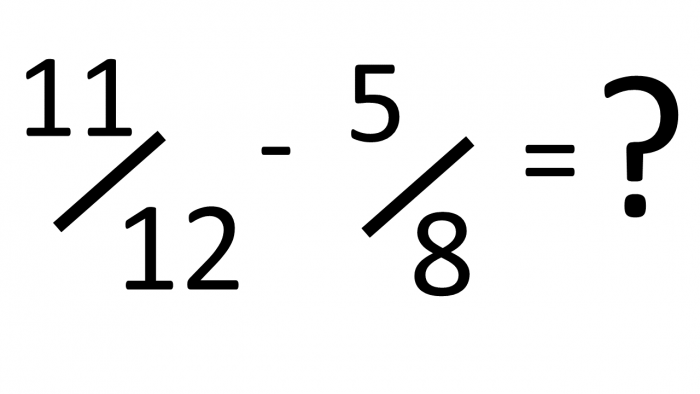102/104

104/152

152/104

144/152

Now, we have the answer for the following calculation, can you choose the correct answers that simplify it to a mixed number?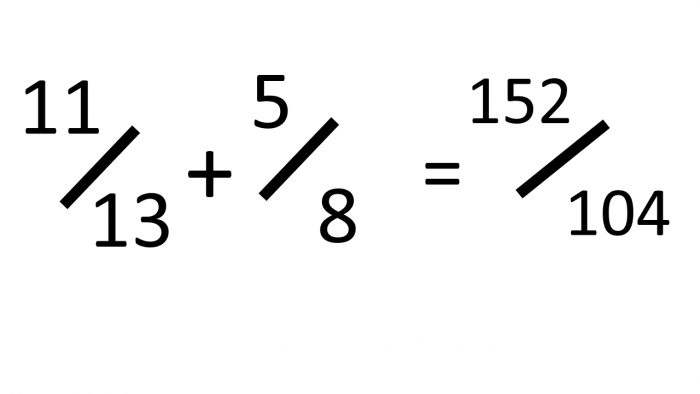1 48 ⁄104

1 48 ⁄48

1 104 ⁄104

1 6 ⁄13

Can you choose the correct answer that represents the mixed number and fractions added together?Can you choose the correct answer that represents the mixed numbers and fractions added together?Can you write the answer to the equation below?Choose your answer by combining a number in the left column with a number from the right.

Select the correct combination below to answer the sum.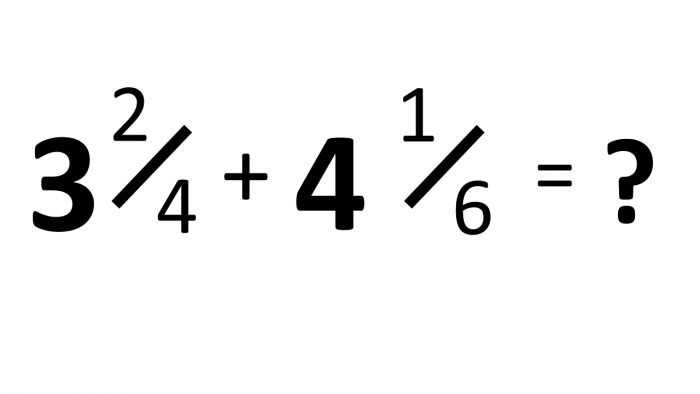Ben and his son went fishing. Ben caught 3 ¾ kg of fish, his son caught 2 ⅕ kg of fish.

What is the total weight of fish they caught?

Complete the blank space.

Write the fraction using '/' and without leaving a space.Mark ran 6 &frac16; km and Reuben ran 1 &frac23; km.

What is the total distance they ran altogether?

Complete the blank space.

Write the fraction using '/' and without leaving a space.

• Question 111/12
EDDIE SAYS
The lowest common multiple of 3 and 4 is 12. This then gives us 8/12 + 3/12 = 11/12.
• Question 213/90
EDDIE SAYS
The lowest common multiple of 10 and 9 is 90. This gives, 63/90 - 50/90 = 13/90
• Question 3

Can you choose the correct answer for the following calculation?152/104
EDDIE SAYS
The lowest common multiple of 13 and 8 is 104. 88/104 + 65/104 = 152/104 The answer is an improper fraction as the numerator is of greater value than the denominator.
• Question 4

Now, we have the answer for the following calculation, can you choose the correct answers that simplify it to a mixed number?1 48 ⁄104
1 6 ⁄13
EDDIE SAYS
When converted to a mixed number, 152/104 is initially changed to 1 and 48/104, this can then be further simplified to 1 and 6/13. The highest common factor of 48 and 104 is 8, so we divide each by 8 to create 6/13. Hopefully, this is beginning to make sense.
• Question 5

Can you choose the correct answer that represents the mixed number and fractions added together?EDDIE SAYS
How did you find this one? First, there was only one whole number, 2, so there was nothing to add. Second, find the same denominator = 84 as it's the lowest common multiple; 24 ⁄ 84 + 48/84 = 72/84 Finally, reduce 72 ⁄ 84 = 6/7 The highest common factor is 12 This leaves us with a final answer of 2 6/7
• Question 6

Can you choose the correct answer that represents the mixed numbers and fractions added together?EDDIE SAYS
Again, we need to follow the same sequence of steps: First, we add the whole numbers together, 2 + 1 = 3. Next, find the same denominator = 12, as it's the lowest common multiple; 3/12 + 2/12 = 5/12 Next, 5 ⁄ 12 cannot be reduced. Finally, add the whole number and fraction together. This leaves us with a final answer of 3 5/12. Don’t panic if you found this difficult, just work through each step! Great focus. You're past halfway...
• Question 7

Can you write the answer to the equation below?Choose your answer by combining a number in the left column with a number from the right.

EDDIE SAYS
1st step: Add the whole numbers together, 1 + 3 = 4 2nd step: Add fractions together by finding the common denominator, the lowest common multiple, which is 15. 3/15 + 10/15 = 13/15 Finally, add the whole numbers and fractions together to give the final answer = 4 13/15
• Question 8

Select the correct combination below to answer the sum.EDDIE SAYS
1st step: Add the whole numbers together, 3 + 4 = 7. 2nd step: Add fractions together by finding the common denominator, the lowest common multiple, which is 12. 6 ⁄12 + 2 ⁄12 = 8 ⁄12. 3rd step: Add whole numbers and fractions together to give the final answer = 7 8/12
• Question 9

Ben and his son went fishing. Ben caught 3 ¾ kg of fish, his son caught 2 ⅕ kg of fish.

What is the total weight of fish they caught?

Complete the blank space.

Write the fraction using '/' and without leaving a space.EDDIE SAYS
This worded problem required us to add 3 3/4 and 2 1/5 together. We had to find the common denominator to add the fractions, which is 20. This gave us 15/20 + 4/20= 19/20. Then added with the whole numbers gave a total of 5 19/20 kg.
• Question 10

Mark ran 6 &frac16; km and Reuben ran 1 &frac23; km.

What is the total distance they ran altogether?

Complete the blank space.

Write the fraction using '/' and without leaving a space.

EDDIE SAYS
This worded problem required us to add 6 1 ⁄6 and 1 4 ⁄6 together. We had to find the common denominator to add the fractions, which is 6. This gave us 1/6 + 4/6 = 5/6. Then added with the whole numbers gave a total of 7 5/6 km. Practice makes perfect! That’s another activity ticked off!
---- OR ----

Sign up for a £1 trial so you can track and measure your child's progress on this activity.

### What is EdPlace?

We're your National Curriculum aligned online education content provider helping each child succeed in English, maths and science from year 1 to GCSE. With an EdPlace account you’ll be able to track and measure progress, helping each child achieve their best. We build confidence and attainment by personalising each child’s learning at a level that suits them.

Get started